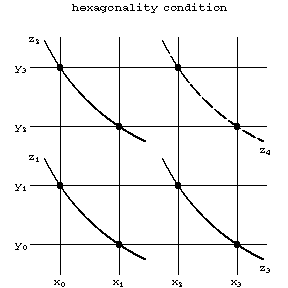# Closure condition

The condition in the geometry of webs (cf. Webs, geometry of) according to which certain incidences of points and lines of a web imply new incidences. For example, Thomsen's closure condition (see Fig.a) is as follows. The first and the second family of lines of a web are represented by parallel straight lines, the third by curves; the closure condition implies that if $A,B,C,D$ lie on curves of the third family, then so do $E$ and $F$.Figure: c022610a

If $x,y$ and $z$ are the parameters defining the lines of the three families, the closure condition can be presented in abstract form as a system of defining equations, regarding $z$ as the "product" of $x$ and $y$: $z=xy$,

$$x_0y_1=x_1y_0,x_0y_2=x_2y_0\succeq x_1y_2=x_2y_1.$$

If $z=xy$ is regarded as a quasi-group operation, Thomsen's closure condition is equivalent to the condition that the quasi-group be isotopic to an Abelian group.Figure: c022610bFigure: c022610c

Fig.b and Fig.c illustrate the Reidemeister closure condition and the hexagonality condition (all three conditions are equivalent for a plane three-web, even without a differentiability assumption). In abstract form these conditions yield different classes of quasi-groups and loops; in the multi-dimensional geometry of webs, they yield different classes of webs.

Some theorems in projective geometry are essentially closure conditions (such as the theorems of Desargues and Pappus).